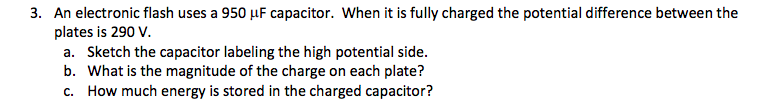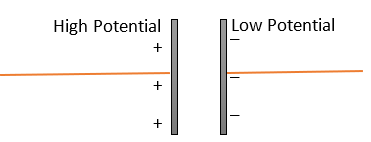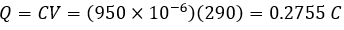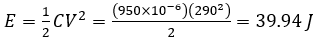# 3. An electronic flash uses a 950 µF capacitor. When it is fully charged the potential difference between theplates is 290 V.a. Sketch the capacitor labeling the high potential side.b. What is the magnitude of the charge on each plate?How much energy is stored in the charged capacitor?

Question
136 viewshelp_outlineImage Transcriptionclose3. An electronic flash uses a 950 µF capacitor. When it is fully charged the potential difference between the plates is 290 V. a. Sketch the capacitor labeling the high potential side. b. What is the magnitude of the charge on each plate? How much energy is stored in the charged capacitor? fullscreen
check_circle

Step 1

Given information:

The capacitance of the capacitor (C) = 950 µF

The potential across the capacitor (V) = 290 V

The capacitor can be picturized as:Step 2

The charge on the capacitor is given by:The energy stored in the capacitor is given by:...

### Want to see the full answer?

See Solution

#### Want to see this answer and more?

Solutions are written by subject experts who are available 24/7. Questions are typically answered within 1 hour.*

See Solution
*Response times may vary by subject and question.
Tagged in